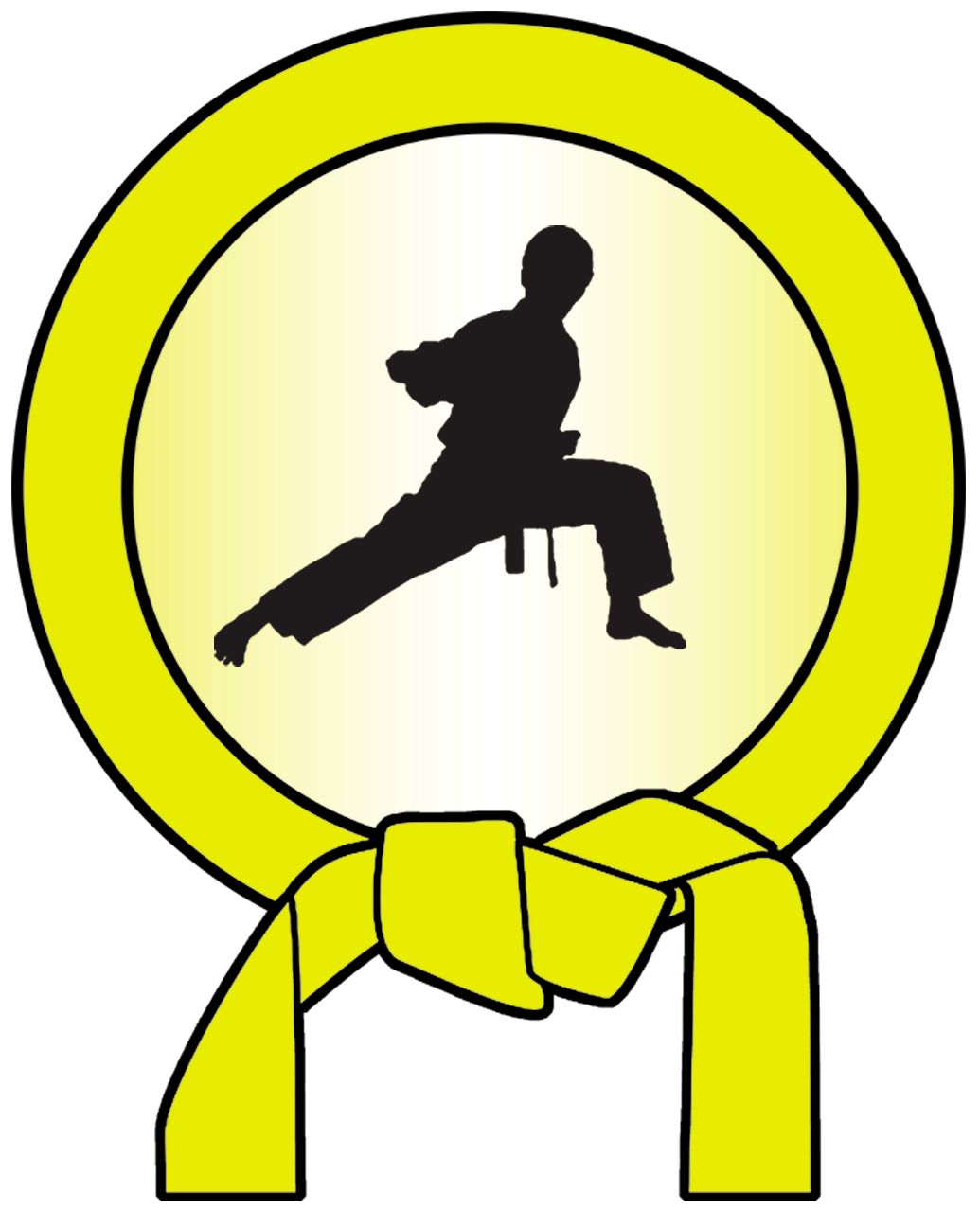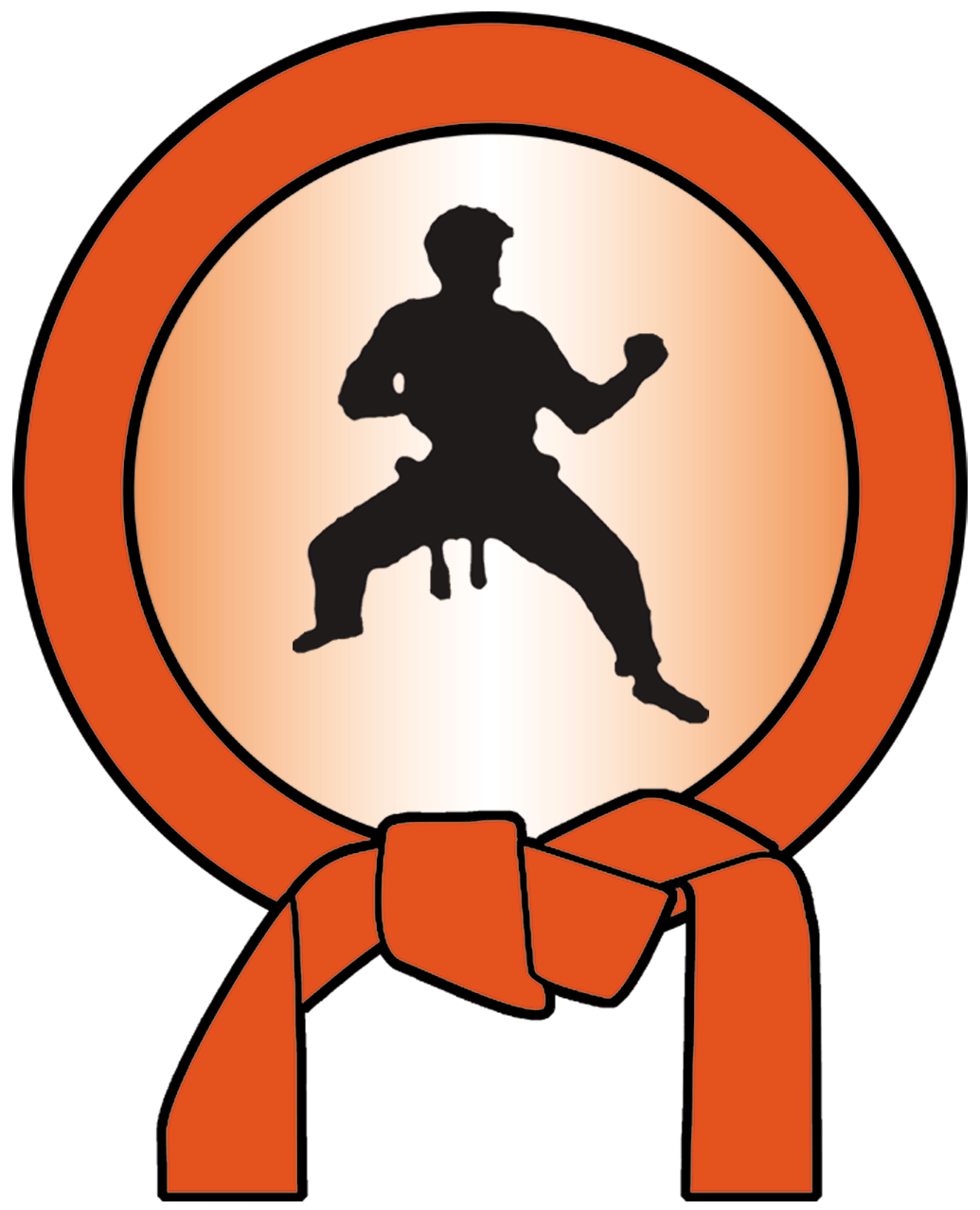# Common Core

We have Common Core Aligned curriculum for 6th, 7th and 8th grades. Each includes open ended problems, projects and activities to help transition to the new smarter balanced assessments.

In Judo Math, each discipline is basically a unit, with four belts of progression. There is also a green sensei belt for the faster paced students to challenge themselves.

### Want to see a sample of our curriculum?NUMBER SENSE (6NS1-7) Ratios  (6RP1-3) Equations/Geom(6EE5-9, 6G1-4)Positive and Negative Rational numbers Opposites Cartesian Coordinates Ratio Ratio tables Double number lines Tape Diagrams Solving Equations Substitution Variables as unknowns Variables as a setAbsolute Value Distance from zero On coordinate plane Real world statements Rate/Percent Unit rates Rates in the world Percent Equations in a given form X + p = q Xp = q Constraint equationsDecimals and long division Add/subtract Multiply/Divide Expression basics Exponents Intro to variables Basics of expressions Area Right triangles Base and height Quadrilaterals polygonsFractions Whole number division Fraction to fraction division Applications Simplifying Expression Combining terms Order of operations/Prop. Simplifying expressions Volume/Surface Area Volume of prism Volume formula Intro to nets Using nets for surface area
 RATIONAL NUMBERS(7NS1-3, 7RP 1) PROBLEM SOLVING (7RP2-3, 7EE1-4) GEOMETRY (7G1-6)Properties Add/Sub on number line Identity, Associative Inverse Proportions Determining proportionality In graphs and equations Interest, tax, percent change Scale What is scale Scaling up/down Reproduce drawingMultiplying Rules for signs Distributive Commutative Linear Expressions Combine like terms Expanding and factoring Rewriting Constructions/angles Drawing shapes Investigating triangles Complimentary/supplim. Solving for anglesFractionology Fraction sense Add/Sub/Mult/Div complex fractions Equations Converting and writing Solve two step Solve multi-step Area 2D vs 3D Circle vocab Pi Area of circles, polygons, irregularsRates Ratios with fractions Unit rate applications Inequalities Graphing Solve two step Solve multi-step Surface Area and Volume Volume and surface area 1-2 Volume and surface area applications
 PROBLEM SOLVING (8NS1-2, 8EE1-4, 7-8, 8SP1-2) FUNCTIONS (8EE5-8, 8F1-5, 8SP3-4) GEOMETRY (8G1-9)Basics Integers Rational vs irrational Evaluate Combining Fundamentals Function vs relations Calculating slope Meaning of slope Transformations Rotations Reflections TranslationsRadicals and Exponents Negative exponents Square and cube root Scientific notation Graphing Data tables Y = mx + b Writing linear equations Congruence/Similarity Using coordinates and transformations to show congruence Congruence vs similaritySolve Linear Equations Linear equations 0, 1, 2 solutions Complex linear equations Identity vs No Solution Modeling Line of best fit Dependent vs Independent variables Algorithms Volume and Angles Angle sum of triangles Exterior angles Parallel lines and transversals Volume of cone, cylinder, sphereApplications Converting Rates D=RT Scatter plots Bivariate data Systems Graphing Solving methods Supply and Demand Pythagorean Theorem Development Proof Problems Showing distance